# Fraction to Percent Calculator

## Convert any fraction to percentage value with just one click.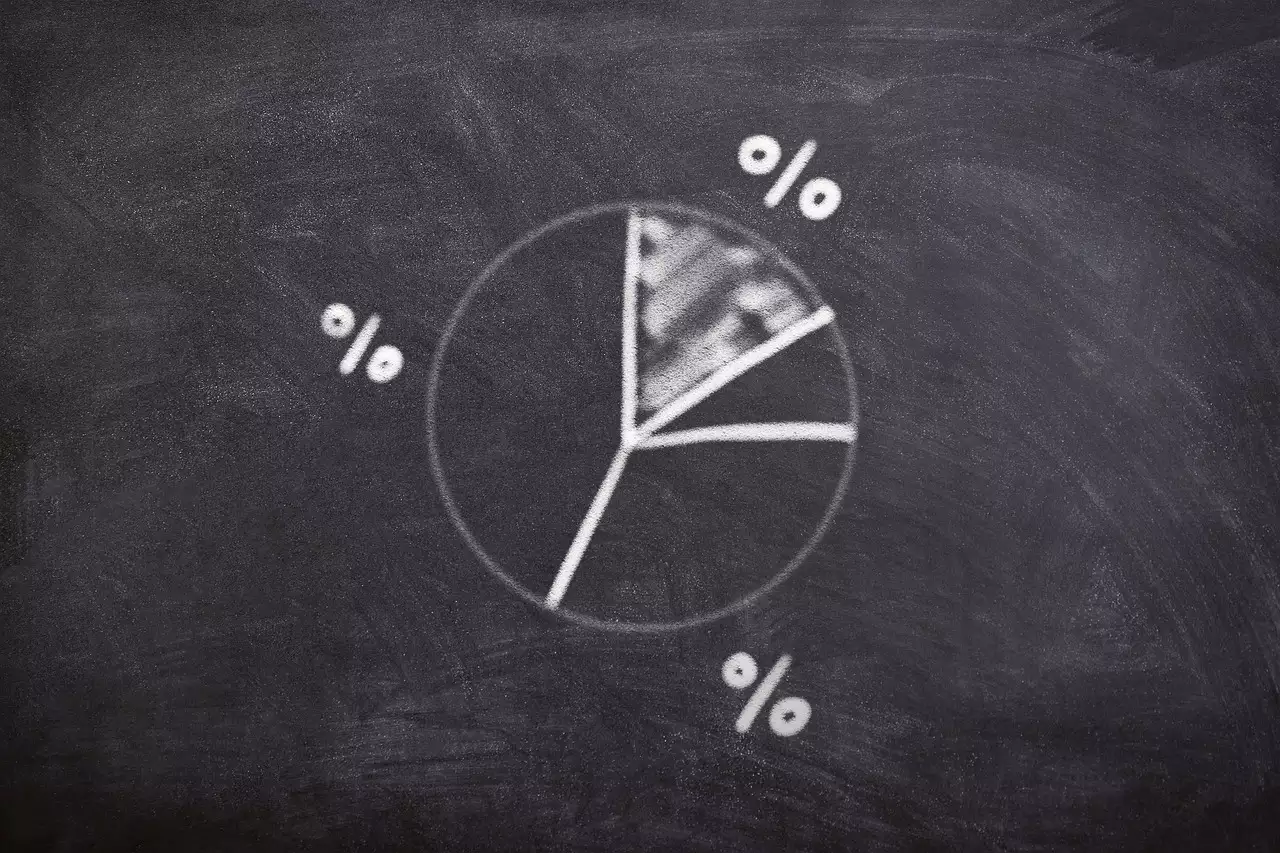A fraction-to-percent calculator is an online tool developed by Calcopolis to help you convert fractions to percents. It's mainly used to convert two types of fractions into percents, which are:

• Proper fractions
• Improper fractions

In a nutshell, proper fractions are those that have a numerator that's less than the denominator (ex: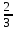). On the other hand, improper fractions have a numerator that's greater in value than the denominator (ex: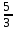).

Tip. If you wish to reverse this operation, use our percent to fraction calculator, which can turn any percentage value into a proper fraction. The tool can simplify fractions to make the result more readable.

## Fractions vs. Percents: A Brief Comparison

Before diving into the basic methods of converting a fraction to a percent, let's go over both of their exact definitions. This will provide you with a better understanding of the steps involved in the conversion process.

### Fractions

Simply put, a fraction represents the parts of a whole and is expressed as a ratio of two numbers.

The numerator (top number) indicates the number of equal parts that are taken from the whole. The denominator (bottom number) shows the total number of equal parts into which the whole is divided.

For example, if a pizza is divided into 7 equal slices, each plate with a slice will have a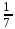of the pizza.

### Percents

A percentage in mathematics is a ratio or number that can be described as a fraction of 100. To calculate a percent of a number, we divide the value by the whole and then multiply the result by 100.

This clarifies the literal meaning of percent, the abbreviation of the Latin phrase "per centum," which translates to "per 100." Now, we have the symbol "%" to express the word percent."

In order to operate on fractions with ease, it is essential to understand the concept of the Greatest Common Factor and Least Common Denominator. Both are described in detail at Calcopolis with simple tools that allow you to calculate these values.

## How to Turn a Fraction Into a Percentage?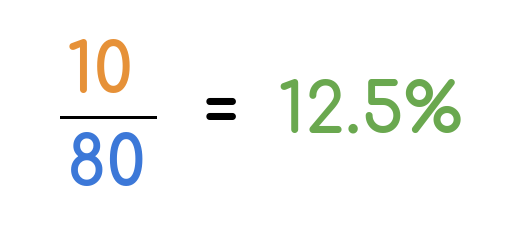With the above definitions in mind, let's look at how a fraction can be tuned to a percent. There are primarily two methods to accomplish this conversion.

Both techniques are straightforward and can be used in any context; they're not limited to specific situations.

Below, you can see examples of how to convert the fraction to percentages.

### 1st Method

The first method is initiated by dividing the numerator by the denominator. That is, to get the decimal equivalent of this fraction. The resulting decimal is then multiplied by 100 to obtain the required "per 100," a.k.a. percent.

#### Example: Convert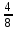to a Percent

1. Divide the numerator 4 by the denominator 8:
4 / 8 = 0.5
2. Multiply the decimal by 100:
0.5 * 100 = 50

### 2nd Method

The second method involves multiplying the fraction's numerator by 100 and then dividing the result by the denominator.

#### Example: Convert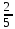to a Percent

1. Multiply the numerator 2 by 100:
2 * 100 = 200
2. Divide the result by the denominator 5:
200 / 5 = 40

## Benefits of Presenting Fractions as a Percent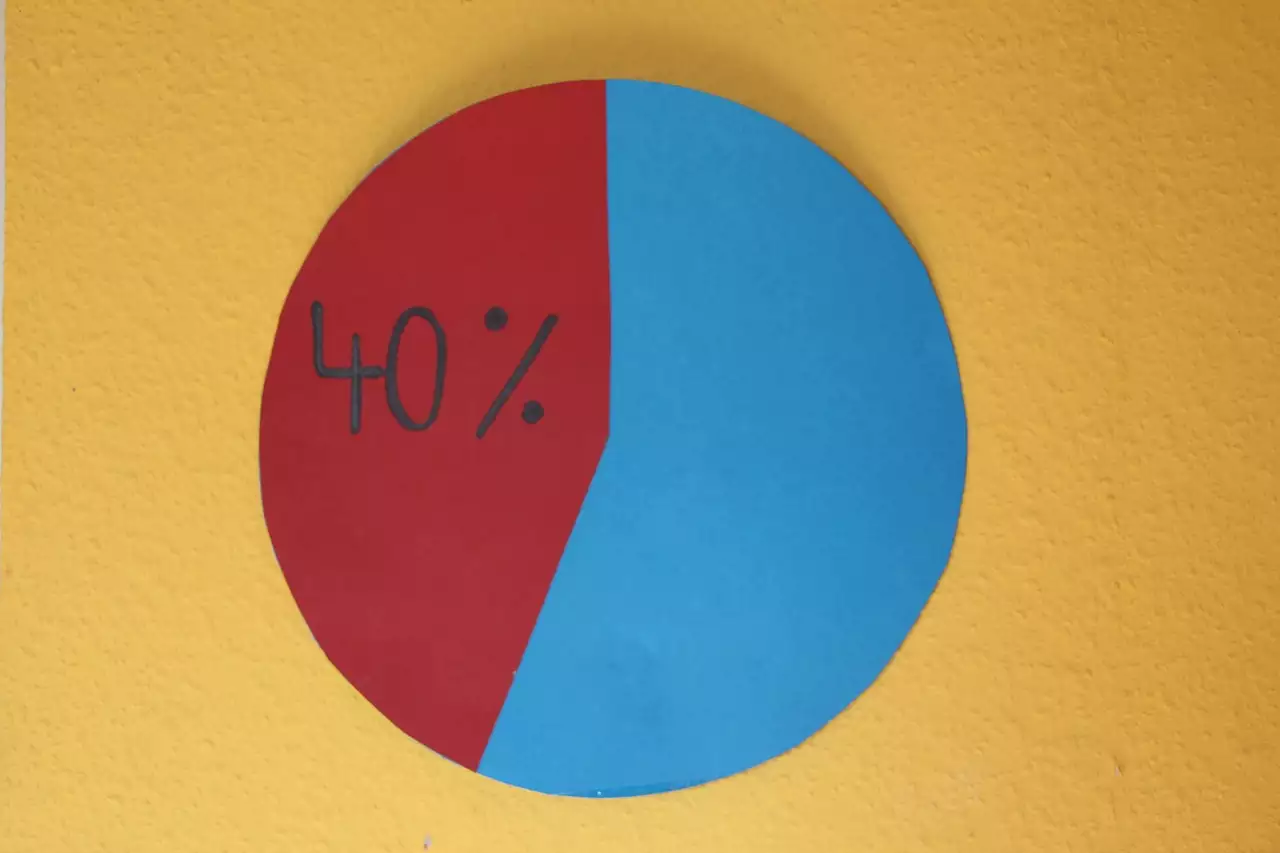Percents help us better understand many aspects of our daily finances. That's one of the reasons why they're heavily relied upon in various industries.

Converting fractions to percents is sometimes necessary to make a figure more digestible. In fact, here are four advantages to expressing fractions as percents:

1. Provide a more descriptive insight when used in presentations and reports
3. Determine the exact tax amount paid for meals at restaurants
4. Compare two or more values in a much simpler form

Example: Comparing males to females ratios in a university is easier when expressed as 60% females to 40% males rather than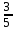females tomales.

## Similar tools

Calcopolis provides many more useful tools that assist you with your daily math calculations. If you wish to turn a fraction into a decimal value, use a fraction to decimal converter, which can turn a fraction into a decimal number with the required precision.

Visit the category page to see all the math tools available on our website. Using Calcopolis regularly can avoid many mistakes in your daily calculations.

### Authors

Created by Lucas Krysiak on 2022-12-21 16:53:53 | Last review by Mike Kozminsky on 2022-12-21 12:14:20